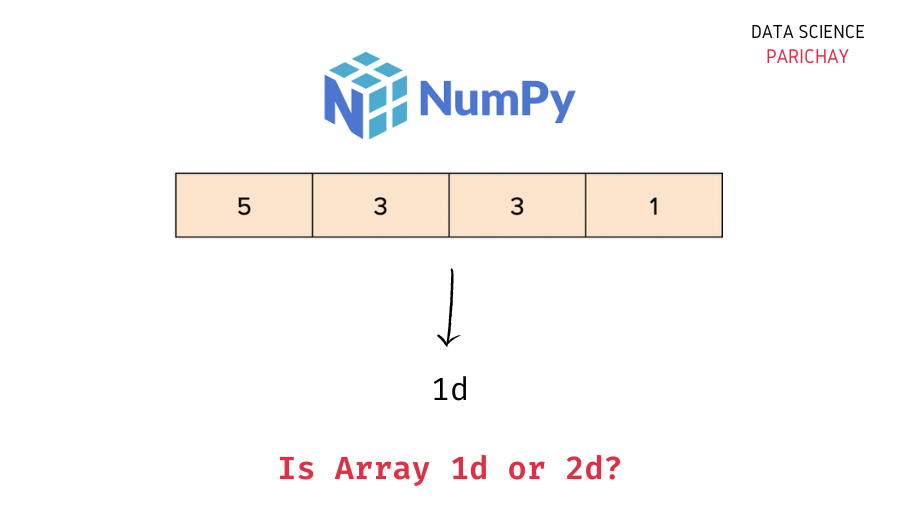# Numpy – Check If Array is 1d or 2d

In this tutorial, we will look at how to check if a numpy array is 1d (one-dimensional), 2-d (two-dimensional), or a higher-dimensional array with some examples.

## How to get the dimensions of a numpy array?

You can use a numpy array’s `ndim` property to get the number of dimensions in the array. For a 1d array, it returns 1, for a 2d array it returns 2, and so on.

The following is the syntax –

```# get the dimensions of a numpy array
df.ndim```

Alternatively, you can also use the `shape` property of a numpy array to determine the dimensionality of an array. It returns a tuple with each element representing the length of the respective dimension in the array.

Let’s now look at some examples of using the above syntax –

## Example 1 – Check if array is 1d, 2d, or a higher dimensional array using `ndim` property

Let’s create a 1d array, a 2d array, and a 3d array and see what we get with the `ndim` property for each of these arrays.

```import numpy as np

# create a 1d array
ar1 = np.array([1, 2, 3])
# create a 2d array
ar2 = np.array([[1, 2, 3], [4, 5, 6]])
# create a 3d array of zeros with 2 rows, 2 columns, and 3 depth
ar3 = np.zeros((2, 2, 3))

# get the dimensions of the arrays
print(ar1.ndim)
print(ar2.ndim)
print(ar3.ndim)```

Output:

```1
2
3```

We get the correct result. You can similarly use the `ndim` property to determine the dimensionality of a numpy array for higher dimensional arrays as well.

📚 Data Science Programs By Skill Level

Introductory

Intermediate ⭐⭐⭐

🔎 Find Data Science Programs 👨‍💻 111,889 already enrolled

Disclaimer: Data Science Parichay is reader supported. When you purchase a course through a link on this site, we may earn a small commission at no additional cost to you. Earned commissions help support this website and its team of writers.

## Example 2 – Using the `shape` property

A numpy array’s `shape` property also gives information about the dimensions in the array. Let’s take the same arrays used in the above example.

```import numpy as np

# create a 1d array
ar1 = np.array([1, 2, 3])
# create a 2d array
ar2 = np.array([[1, 2, 3], [4, 5, 6]])
# create a 3d array of zeros with 2 rows, 2 columns, and 3 depth
ar3 = np.zeros((2, 2, 3))

# get the shape of the arrays
print(ar1.shape)
print(ar2.shape)
print(ar3.shape)```

Output:

```(3,)
(2, 3)
(2, 2, 3)```

The `shape` returns a tuple with the number of values in each dimension of a numpy array. For `ar1` we only get a single value as there’s only one dimension in the array. Thus, you can determine the dimensions by calculating the length of the `shape` tuple.

```# get the dimensions of the arrays
print(len(ar1.shape))
print(len(ar2.shape))
print(len(ar3.shape))```

Output:

```1
2
3```

We get the same results as above.

You might also be interested in –

•